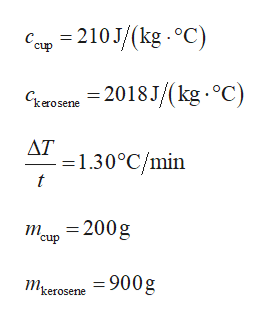# A tin calorimeter cup with a specific heat of 210 J/(kg · °C)contains kerosene with a specific heat of 2,018 J/(kg · °C) at 16.0°C. The combination is heated uniformly so that the temperature changes by 1.30°C per minute. If the cup has a mass of 200 g and contains 900 g of the liquid, determine the rate at which heat energy is added (in W).

Question
39 views

A tin calorimeter cup with a specific heat of 210 J/(kg · °C)contains kerosene with a specific heat of 2,018 J/(kg · °C) at 16.0°C. The combination is heated uniformly so that the temperature changes by 1.30°C per minute. If the cup has a mass of 200 g and contains 900 g of the liquid, determine the rate at which heat energy is added (in W).

check_circle

Step 1

Givenhelp_outlineImage TranscriptioncloseСар 3 210J/(kg.°C) с. сup -2018 J / (kg.C Сквовете ДТ -1.30°C/min t = 200g т cup тerоsene 900g kerosene fullscreen
Step 2

The rate of heat ...

### Want to see the full answer?

See Solution

#### Want to see this answer and more?

Solutions are written by subject experts who are available 24/7. Questions are typically answered within 1 hour.*

See Solution
*Response times may vary by subject and question.
Tagged in

### Heat Transfer